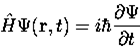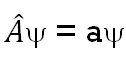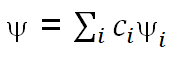Goseeko blog# What are postulates of Quantum Mechanics?

## Quantum Mechanics

Max Born in 1924 coined the term “quantum mechanics”.

Quantum mechanics is the branch of physics dealing with the behaviour of matter and light on the atomic and subatomic level. Thus it attempts to describe and account for the properties of molecules and atoms. And their constituents—electrons, protons, neutrons, and other more particles such as quarks and gluons.

According to classical mechanics objects exist in a particular place at a  particular time. However, according to quantum mechanics, objects instead exist in a haze of probability. They have a probability of being at point A and another probability of being at point B and so on. It results in some very strange conclusions about the physical world.

At the turn of the twentieth century, however, classical physics was not able to explain some of the phenomena. Hence quantum mechanics explain those  phenomena.

## Postulates

#### Postulate 1

The state of a quantum mechanical system is completely specified by a wave function ψ(r,t). This function is the wave function or state function. Wave function ψ(r,t) depends on the coordinates of the particle and on time.

Wave function or state function has the important property that the square of the wave function gives the probability density of the particle. The quantity |ψ(r,t)|2 is called the probability. And this quantity simply means probability of finding the particle at position at any instant of time.

The wave function must also be single-valued, finite and continuous. Because of the probabilistic interpretation, the wave function must satisfy certain mathematical conditions. For the case of a single particle, the probability of finding is 1. From here we have got normalization condition.

#### Postulate 2

To every observable we deal in classical mechanics, there exists a corresponding linear and Hermitian operator in quantum mechanics. For example, to momentum px  there exists corresponding momentum operator  in the direction for a single particle is –iℏ∂∂x.

#### Postulate 3

Corresponding to a operator, the average value of the observable is#### Postulate 4

According to the time-dependent Schrödinger equation the wave unction evolves in time.#### Postulate 5

For any measurement of the observable quantity associated with the operator. Thus  the values that will be calculated are the eigenvalues.  Eigenvalues satisfy the following equation.Although calculation must always yield an eigenvalue. However, the state does not originally have to be in an eigen state of an operator. Thus an arbitrary state can expand in the complete set of eigenvectors of operator aswhere the sum can go to infinity in principle. The probability of observing eigenvalue ai is given by ci*ci.

#### Postulate 6

The total wave function must be anti-symmetric with respect to the interchange of all coordinates of one fermion with those of another. However, Electronic spin must be taken into account. Also the Pauli Exclusion Principle is a direct result of this anti-symmetry principle.

Interested in learning about similar topics? Here are a few hand-picked blogs for you!